# Millman’s Theorem

## Network Analysis Techniques

• #### Question 1

 Don’t just sit there! Build something!!

Learning to mathematically analyze circuits requires much study and practice. Typically, students practice by working through lots of sample problems and checking their answers against those provided by the textbook or the instructor. While this is good, there is a much better way.

You will learn much more by actually building and analyzing real circuits, letting your test equipment provide the “answers” instead of a book or another person. For successful circuit-building exercises, follow these steps:

1. Carefully measure and record all component values prior to circuit construction.
2. Draw the schematic diagram for the circuit to be analyzed.
3. Carefully build this circuit on a breadboard or other convenient medium.
4. Check the accuracy of the circuit’s construction, following each wire to each connection point, and verifying these elements one-by-one on the diagram.
5. Mathematically analyze the circuit, solving for all values of voltage, current, etc.
6. Carefully measure those quantities, to verify the accuracy of your analysis.
7. If there are any substantial errors (greater than a few percent), carefully check your circuit’s construction against the diagram, then carefully re-calculate the values and re-measure.

Avoid very high and very low resistor values, to avoid measurement errors caused by meter “loading”. I recommend resistors between 1 kΩ and 100 kΩ, unless, of course, the purpose of the circuit is to illustrate the effects of meter loading!

One way you can save time and reduce the possibility of error is to begin with a very simple circuit and incrementally add components to increase its complexity after each analysis, rather than building a whole new circuit for each practice problem. Another time-saving technique is to re-use the same components in a variety of different circuit configurations. This way, you won’t have to measure any component’s value more than once.

• #### Question 2

Convert all the “Thévenin” sources into Norton equivalent sources in this network: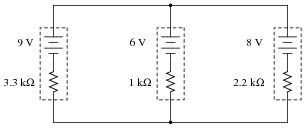• #### Question 3

Simplify this circuit by combining all Norton sources into one, then solve for the voltage between the two busses: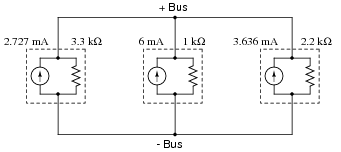• #### Question 4

Write an algebraic equation that solves for the voltage between the two bus conductors, based on the problem-solving method of Thévenin-to-Norton conversion, combining Norton sources into one, and combining resistors into one: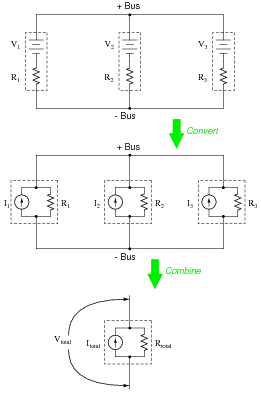• #### Question 5

Calculate the voltage indicated by the voltmeter in this circuit for the following voltage inputs: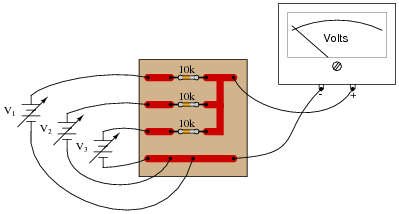V1 = 4.0 volts
V2 = 5.0 volts
V3 = 12.0 volts

What do you notice about the output voltage of this circuit? What mathematical function does this circuit perform?

• #### Question 6

Suppose this circuit was found to output a voltage of 11.0 volts, given the input voltages shown: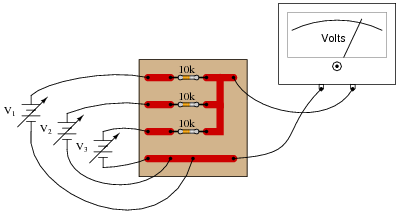V1 = 8.5 volts
V2 = 10.0 volts
V3 = 12.0 volts

What do suspect is wrong with this circuit? How would you verify the cause of the incorrect output voltage?

• #### Question 7

What would a digital voltmeter register, if connected to the circuit as shown below?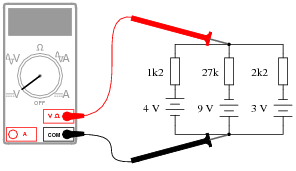• #### Question 8

A set of batteries are connected in parallel to form a battery bank. Ideally, their individual voltages would be exactly equal, and there would be no stray resistance anywhere in the circuit, but in reality what we have is something like this:Use Millman’s theorem to calculate the total voltage between the two busses for the battery bank, given these specifications for the four batteries:

 Battery Voltage Rconnection Rconnection − Rinternal 1 11.9 1.2 Ω 1.1 Ω 5.5 Ω 2 12.2 1.0 Ω 1.3 Ω 5.1 Ω 3 12.0 1.4 Ω 0.9 Ω 4.7 Ω 4 12.1 1.1 Ω 1.2 Ω 5.5 Ω

• #### Question 9

Calculate the voltage across the starter motor terminals of the “dead” car, and the current through the starter motor, while a second car is giving it a jump-start: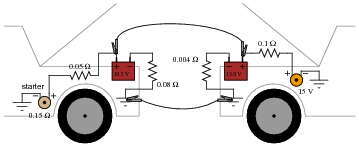Regard the starter motor itself as a 0.15 Ω resistor, and disregard any resistance of the jumper cables connecting the two cars’ electrical systems together.